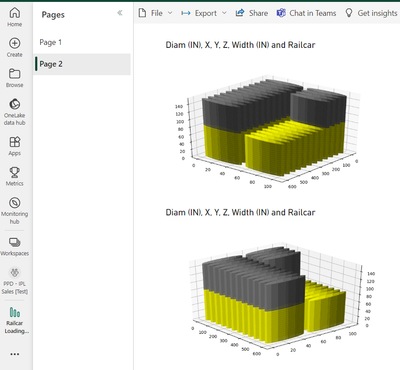cancel
Showing results for
Did you mean:

Fabric is Generally Available. Browse Fabric Presentations. Work towards your Fabric certification with the Cloud Skills Challenge.

## Python visual on the Service doesn't render as in PBI Desktop

The error message I get on the Service is:

"It is not currently possible to manually set the aspect on 3D axes"

But it rendered normally on Desktop (see below).

The method causing the issue is set_x/y/z/ticks from Matplotlib : ax.set_yticks(np.arange(0, 108, step=25))

Is there any timeline for fixing this issue or workaround? I do need to plot it as a rectangle. A square won't look good.Status: Needs Info

Is this the first time you've uploaded a visual of this type? Have you had similar problems before? What is your data source? What is your Desktop version ?

Best Regards,
Community Support Team _ Ailsa TaoCommunity Support
Status changed to: Needs Info

Is this the first time you've uploaded a visual of this type? Have you had similar problems before? What is your data source? What is your Desktop version ?

Best Regards,
Community Support Team _ Ailsa TaoFrequent Visitor

Hello Alisa

That's the first time I use a Python visual in a professional setting.

Currently, I am pulling data from an Excel file.

PBI Desktop version: 2.121.942.0 64-bit (September 2023)

Please see below that if I comment the lines regarding axis formatting, it works.Please find the viz code below:

``````# The following code to create a dataframe and remove duplicated rows is always executed and acts as a preamble for your script:

# dataset = pandas.DataFrame(Diam (IN), X, Y, Z, Width (IN), Railcar)
# dataset = dataset.drop_duplicates()

# Paste or type your script code here:

df = dataset

import matplotlib.pyplot as plt
from mpl_toolkits.mplot3d import Axes3D
from matplotlib.path import Path
import numpy as np

def plot_cylinder(x, y, z, r, h):

if y == 0:
y = r
elif y > 0:
y = y + r + 5

#color the cylinder
if z == 82:
cylinder_color = 'brown'
elif z == 68:
cylinder_color = 'gray'
elif z == 70:
cylinder_color = 'blue'
elif z == 80:
cylinder_color = 'yellow'
elif z == 82:
cylinder_color = 'purple'
elif z == 85:
cylinder_color = 'yellow'
elif z == 90:
cylinder_color = 'red'
elif z == 102:
cylinder_color = 'green'

z = np.linspace(0, height_z, 50)
theta = np.linspace(0, 2*np.pi, 50)
theta_grid, z_grid=np.meshgrid(theta, z + h)
return x_grid,y_grid,z_grid

Xc,Yc,Zc = data_for_cylinder_along_z(x+r, y, r, z)
ax.plot_surface(Xc,Yc,Zc,
alpha=0.95,
color= cylinder_color,
#cmap='Set1',
rstride=3,
cstride=3
)

fig = plt.figure(figsize=(7,4))
ax = Axes3D(fig)

#ax.set_xlim3d(0, 600)
#ax.set_ylim3d(0, 108)
#ax.set_zlim3d(0, 154)

#ax.set_zticks(np.arange(0, 154, step=10))
#ax.zaxis.set_tick_params(labelsize=5)

#ax.set_yticks(np.arange(0, 108, step=25))
#ax.yaxis.set_tick_params(labelsize=5)

#ax.set_xticks(np.arange(0, 600, step=25))
#ax.xaxis.set_tick_params(labelsize=5)

for i, row in df.iterrows():

plot_cylinder(
int(row['X']),
int(row['Z']),
int(row['Width (IN)']),
int(row['Diam (IN)']) / 2,
int(row['Y'])

)

ax.view_init(30, 40)

plt.show()``````Frequent Visitor

@v-yetao1-msft , do you need any extra information to investigate it?

Completed Ideas